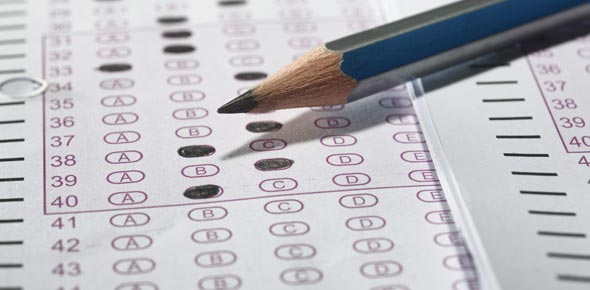# Redox Reactions :D

5 Questions | Total Attempts: 209SettingsA quiz with 5 MCQ questions on redox reactions

Related Topics
• 1.
The reaction between mangananese metal (Mn) and dilute acid is shown by the following ionic equation: Mn(s) + 2H+ (aq) --> Mn2+ (aq) + H2 (g)Which one of the following shows the change in oxidisation state of the hydrogen in this reaction?
• A.

+1 to 0

• B.

0 to +1

• C.

+2 to 0

• D.

+2 to +1

• 2.
Which of the following underlined atoms are reduced?(i)PbCl2 + Cl2 --> PbCl4(ii)NaOH + HCl --> I2 + 5SO42- + 3H + H20(iii)2IO3- + 5HSO3- --> I2 + 5SO42- + 3H+ + H2O(iv)Cu+1/2O2 --> CuO
• A.

I and iv

• B.

Ii and iii

• C.

Iii and iv

• D.

I, ii and iii

• E.

I, ii, iii and iv

• 3.
Which reagent is used to test for oxidising agents?
• A.

Acidified dichromate(VI) solution

• B.

Potassium iodide solution

• C.

Iodine Solution

• D.

Acidified potassium manganate (VII) solution

• 4.
In electrolysis, Oxidisation always takes place
• A.

At the cathode

• B.

At the anode

• C.

At both electrodes

• D.

Between the two electrodes

• 5.
A reducing agent can
• A.

Give oxygen to another substance

• B.

Decrease the oxidisation state of another substance

• C.

Take hydrogen from another substance

• D.

Take electrons from another substance